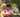### Triangle

January 17, 2020

Triangle

An array A consisting of N integers is given. A triplet (P, Q, R) is triangular if 0 ≤ P < Q < R < N and:
• A[P] + A[Q] > A[R],
• A[Q] + A[R] > A[P],
• A[R] + A[P] > A[Q].

For example, consider array A such that:
A = 10
A = 2
A = 5
A = 1
A = 8
A = 20
Triplet (0, 2, 4) is triangular.

Write a function:
function solution(A);
that, given an array A consisting of N integers, returns 1 if there exists a triangular triplet for this array and returns 0 otherwise.

For example, given array A such that:
A = 10
A = 2
A = 5
A = 1
A = 8
A = 20
the function should return 1, as explained above.

Given array A such that:
A = 10
A = 50
A = 5
A = 1
the function should return 0.

Write an efficient algorithm for the following assumptions:
• N is an integer within the range [0..100,000];
• each element of array A is an integer within the range [−2,147,483,648..2,147,483,647].

``````function solution(A) {
// write your code in JavaScript (Node.js 8.9.4)
A.sort((a, b) => a - b) // ES2015 arrow function callback
//console.log(A)
for(let i=0; i<A.length-2; i++){
if( (A[i] + A[i+1] > A[i+2]) &&
(A[i] + A[i+2] > A[i+1]) &&
(A[i+1] + A[i+2] > A[i])
)
return 1
}
return 0
}``````Posted by Tai Lu ( 呂台生 ) who's building useful things.You should follow him on Twitter